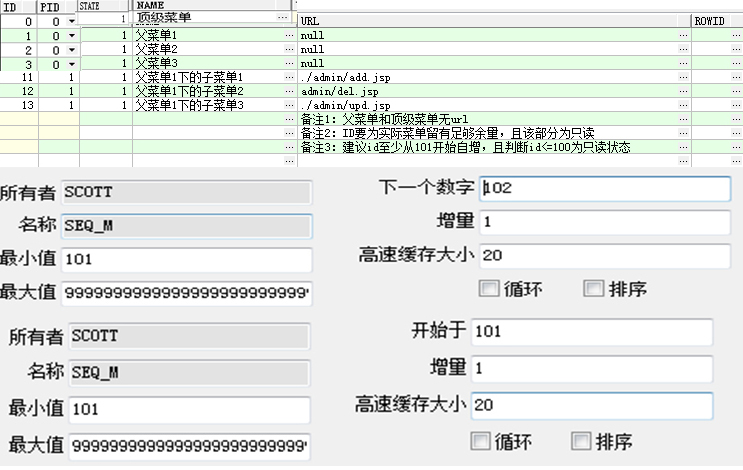详细解读权限控制中的ER关系(新手入门必备知识)

User表实体类：(仅仅写主要部分)

private Integer id;
private String account;
private String name;
private Integer state;
// 多对一:外键r_id
private Role role;

}

Role表实体类:

public class Role {
private Integer id;
private String name;
private Integer state;
// 一对多
// 一对多 中间表mid

}

private Integer id;

private Integer state;
private String name;
private String url;
// 多对一：外键p_id
// 一对多：中间表rid
private Set<Role> roles;

}

ER关系分析的前提是要搞清楚是针对哪个实体对象（Java是面向对象设计的）而言的，如果没有这个前提还大夸奇谈一对一、一对多、多对多关系简直是扯淡，也是很多初学者搞不清的根本原因。

Hibernate中：

<hibernate-mapping package="com.xxx.bean">
<!-- lazy表示懒加载技术 ,inverse指定维护关系，order-by根据某属性排序-->

<!-- 主键 -->
<id name="id" column="id" type="long">
<generator class="sequence">
</generator>
</id>

<set name="roles" lazy="false" inverse="true" table="ROLE_MENU" >
<key column="mid" />
<many-to-many class="Role" column="rid" />
</set>

<!-- 多对一关系用many-to-one 属性parent -->
<many-to-one name="parent" column="p_id" class="Menu" lazy="false" />

<!-- 一对多关系用one-too-many 属性childs -->
<list name="childs" lazy="false" inverse="true" order-by="id">
<key column="p_id" />
</list>

<!-- 其他属性 -->
<property name="name" column="name" />
<property name="url" column="url" />

<property name="status" column="status" />

</class>
</hibernate-mapping>

mybatis中：

mybatis中除了一对一关系，其他所有关系转化为 多对一association或一对多collection关系，二者可嵌套使用，即多对多转化为两个针对不同实体对象的一对多

<mapper namespace="com.salmon.mapper.RoleMapper">
<resultMap type="com.salmon.model.Role" id="role_ID">
<id property="id" column="r_id" />
<result property="name" column="rname" />
<result property="state" column="rstate" />
<id property="id" column="a_id" />
<result property="account" column="account" javaType="java.lang.String" jdbcType="VARCHAR" />
<result property="realname" column="realname" />
<result property="state" column="astate" />
</collection>
<!--   ####      嵌套使用     ####   -->
<id property="id" column="m_id" />
<result property="state" column="mstate" />
<result property="name" column="mname" />
<result property="url" column="url" />
<id property="id" column="pm_id" />
<result property="state" column="pm_state" />
<result property="name" column="pm_name" />
</association>
</collection>
</resultMap>
<!--  注意resultMap中的属性用到了才配，没用到无须配置(多配不用不影响)，且一定要确保包含所有对应的sql查询的结果映射关系；

<!-- 模糊分页查询 -->
<sql id="fuzzy">
<!-- 条件省略-->
</sql>
<select id="fuzzyFindByPage" resultMap="role_ID" parameterType="java.util.Map">
<if test="page!=null">
select * from (
select rownum as rn ,temp.* from (
</if>
select r.id as r_id , r.name as rname,r.state as rstate ,a.id as a_id,a.account ,a.password,a.realname,a.state as astate,
a.rid,m.id as m_id,m.pid,m.state as mstate ,m.name as mname,m.url,pid.id as pm_id,pid.state as pm_state,pid.name as pm_name
 from role r ,admin a,menu m ,role_menu rm ,menu pid where pid.id=m.pid and r.id=rm.rid and m.id=rm.mid and a.rid=r.id
<include refid="fuzzy" />
<if test="page!=null">
) temp
where rownum <![CDATA[<=]]>
#{page.pageNum}*#{page.pageSize}
) tp where tp.rn <![CDATA[>=]]>
(#{page.pageNum}-1)*#{page.pageSize}+1
</if>
</select>
</mapper>